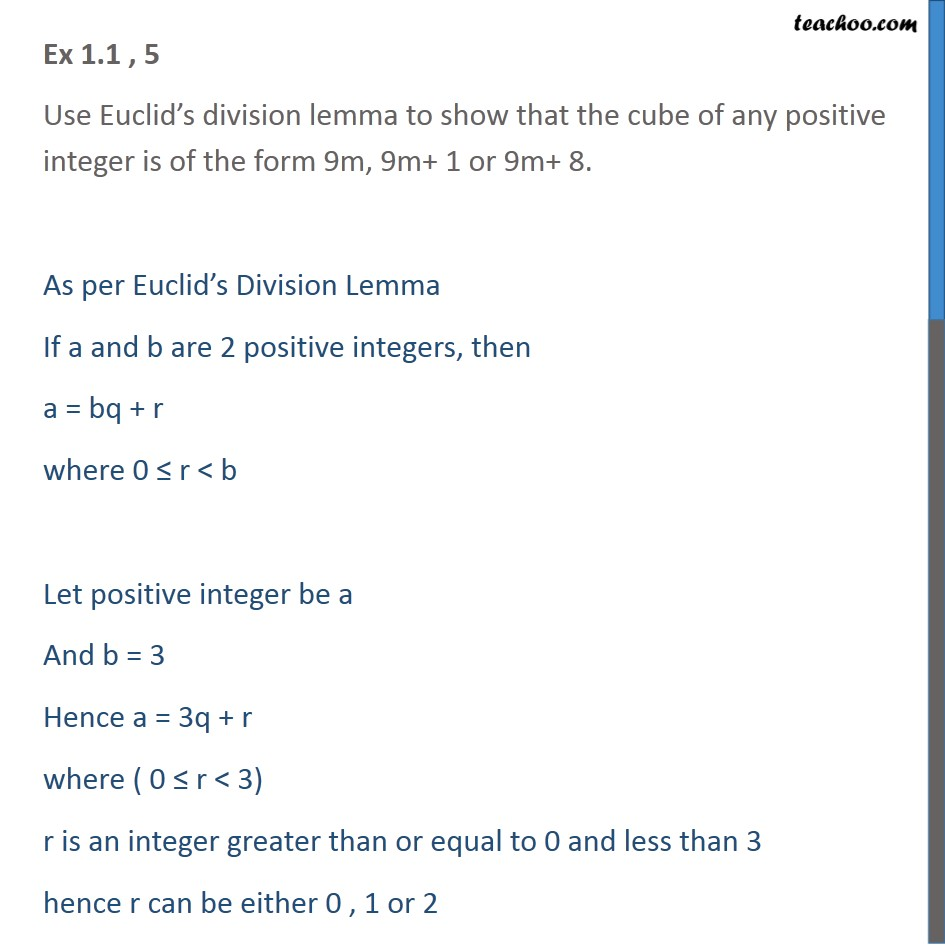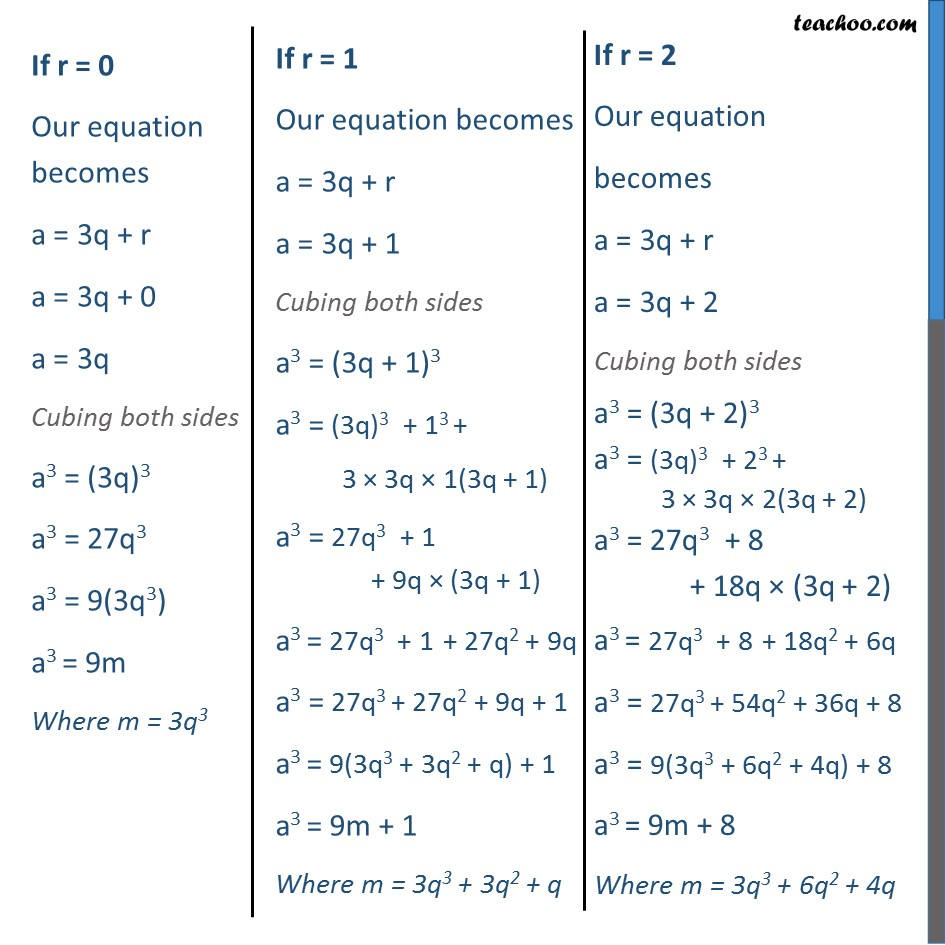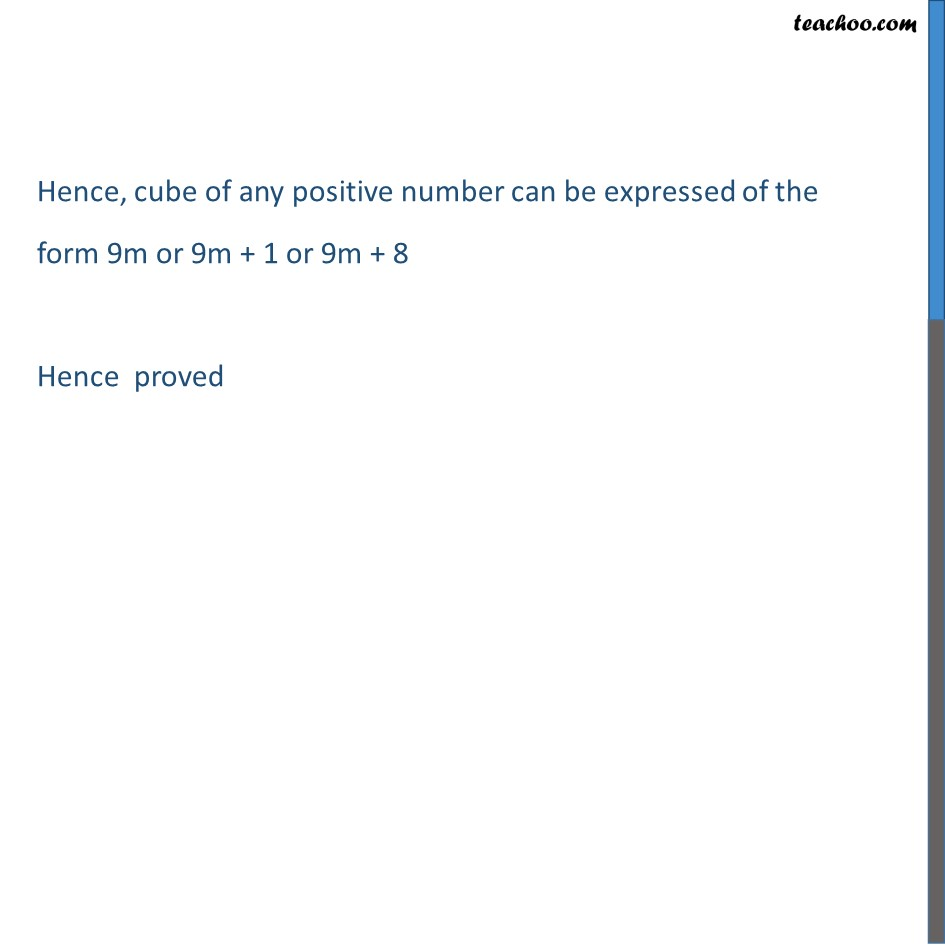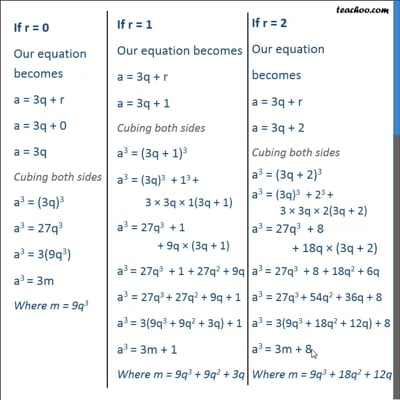Chapter 1 Class 10 Real Numbers

Class 10
Important Questions for Exam - Class 10This video is only available for Teachoo black users

Introducing your new favourite teacher - Teachoo Black, at only ₹83 per month

### Transcript

Ex 1.1 , 5 Use Euclid’s division lemma to show that the cube of any positive integer is of the form 9m, 9m+ 1 or 9m+ 8. As per Euclid’s Division Lemma If a and b are 2 positive integers, then a = bq + r where 0 ≤ r < b Let positive integer be a And b = 3 Hence a = 3q + r where ( 0 ≤ r < 3) r is an integer greater than or equal to 0 and less than 3 hence r can be either 0 , 1 or 2 If r = 0 Our equation becomes a = 3q + r a = 3q + 0 a = 3q Cubing both sides a3 = (3q)3 a3 = 27q3 a3 = 9(3q3) a3 = 9m Where m = 3q3 If r = 1 Our equation becomes a = 3q + r a = 3q + 1 Cubing both sides a3 = (3q + 1)3 a3 = (3q)3 + 13 + 3 × 3q × 1(3q + 1) a3 = 27q3 + 1 + 9q × (3q + 1) a3 = 27q3 + 1 + 27q2 + 9q a3 = 27q3 + 27q2 + 9q + 1 a3 = 9(3q3 + 3q2 + q) + 1 a3 = 9m + 1 Where m = 3q3 + 3q2 + q If r = 2 Our equation becomes a = 3q + r a = 3q + 2 Cubing both sides a3 = (3q + 2)3 a3 = (3q)3 + 23 + 3 × 3q × 2(3q + 2) a3 = 27q3 + 8 + 18q × (3q + 2) a3 = 27q3 + 8 + 18q2 + 6q a3 = 27q3 + 54q2 + 36q + 8 a3 = 9(3q3 + 6q2 + 4q) + 8 a3 = 9m + 8 Where m = 3q3 + 6q2 + 4q Hence, cube of any positive number can be expressed of the form 9m or 9m + 1 or 9m + 8 Hence proved